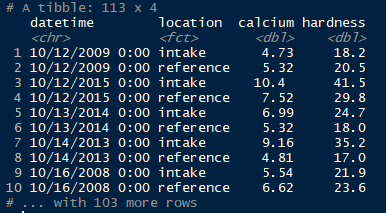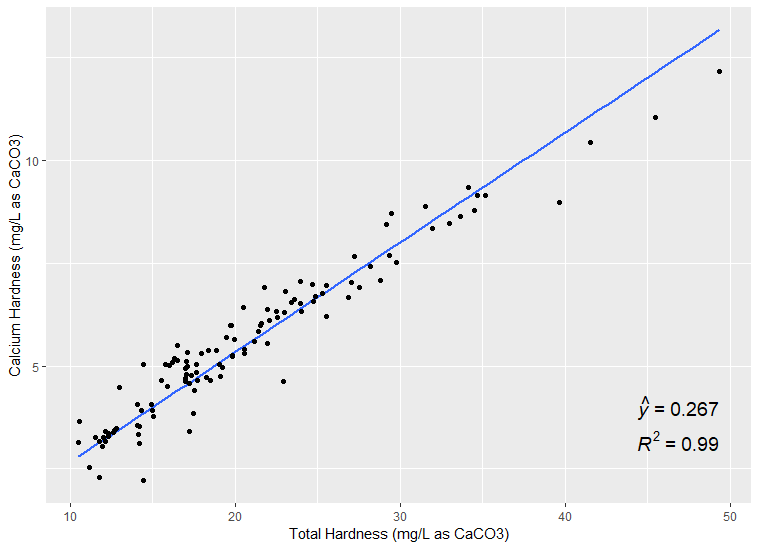# ggpmisc: plotting linear regression equation forced through the origin

Issue #23 resolvedFormer user created an issue

Hello and thanks for your work on ggpmisc!

I ran into a small issue when trying to plot a linear regression equation forced through the origin.

I have a dataframe that looks like this...I'm trying to create a scatterplot of Total Hardness vs. Calcium Hardness and the linear regression equation on the plot with R^2. Using stat_poly_eq() I am very close but can't get the equation quite right.The equation reads "yhat = 0.267" (see above) when it should read "yhat = 0.267x". It works when I don't force the equation through the origin but not when I do. Don't know if this is a bug or my lack of familiarity with the syntax.

Thanks!

## Comments (2)

1.repo owner

Hello,

Please in the future include the code you are using to create the plot together with some example data. The example below shows how the equation is formatted using the version of 'ggpmisc' available here in the repository. I think this should also produce the correct plot with the version of 'ggpmisc' available in CRAN. I will submit the new version to CRAN in coming days.

```library(ggpmisc)
my.df <- data.frame(x = 0:100, y = 0:100 + rnorm(101, sd = 30))
my.formula <- y ~ x - 1
ggplot(my.df, aes(x, y)) +
geom_point() +
geom_smooth(method = lm, formula = my.formula) +
stat_poly_eq(formula = my.formula, aes(label = stat(eq.label)),
eq.with.lhs = "italic(hat(y))~`=`~", parse = TRUE)
```2.repo owner

This was not a bug as far as I can see, but instead a support request. No reproducible code example was provided.

3. Log in to comment